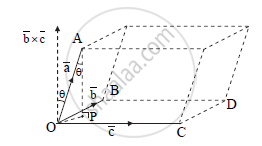# Prove that the Volume of a Parallelopiped with Coterminal Edges as a,b,c Hence Find the Volume of the Parallelopiped with Coterminal Edges i+j, j+k - Mathematics and Statistics

Prove that the volume of a parallelopiped with coterminal edges as   bara ,bar b , barc

Hence find the volume of the parallelopiped with coterminal edges  bar i+barj, barj+bark

#### SolutionLet, bar a, bar b and bar c be the position vectors of points A, B and C respectively with respect to origin O.
Complete the parallelopiped as shown in the figure with  bar(OA) , bar(OB) and bar(OC) as its coterminus edges.
AP is a perpendicular drawn to the plane of bar b and bar c . Let, θ be the angle made by AP with OA.
Volume of parallelopiped = (Area of parallelogram OCDB) x (height)
Now, area of parallelogram OCDB = |barb xxbarc| ….(i)

Height of parallelepiped =l(AP)

=l(OA) cos theta

= |bar(OA)| cos theta

= |bara| cos theta .....(ii)

From (i) and (ii) we get,

volume of parallelepiped =|bara||barbxxbarc|costheta

= bar a.(barb xx barc)

volume of parallelepiped=[bara barb barc]

Let bar a = hati + hatj ,bar b = hatj + hatk , c = hati + hatk

[bar a bar b barc]=|[1,1,0],[0,1,1],[1,0,1]|

=1(1-0)-1(0-1)+0

=1+1

=2

Concept: Scalar Triple Product of Vectors
Is there an error in this question or solution?
2014-2015 (October)

Share